# The Epistemology of Visual Thinking in Mathematics

First published Fri Oct 2, 2015; substantive revision Wed Feb 19, 2020

Visual thinking is widespread in mathematical practice, and has diverse cognitive and epistemic purposes. This entry discusses potential roles of visual thinking in proving and in discovering, with some examples, and epistemic difficulties and limitations are considered. Also discussed is the bearing of epistemic uses of visual representations on the application of the a priori–a posteriori distinction to mathematical knowledge. A final section looks briefly at how visual means can aid comprehension and deepen understanding of proofs.

## 1. Introduction

Visual thinking is a feature of mathematical practice across many subject areas and at many levels. It is so pervasive that the question naturally arises: does visual thinking in mathematics have any epistemically significant roles? A positive answer begets further questions. Can we rationally arrive at a belief with the generality and necessity characteristic of mathematical theorems by attending to specific diagrams or images? If visual thinking contributes to warrant for believing a mathematical conclusion, must the outcome be an empirical belief? How, if at all can visual thinking contribute to understanding abstract mathematical subject matter?

Visual thinking includes thinking with external visual representations (e.g., diagrams, symbol arrays, kinematic computer images) and thinking with internal visual imagery; often the two are used in combination, as when we are required to visually imagine a certain spatial transformation of an object represented by a diagram on paper or on screen. Almost always (and perhaps always) visual thinking in mathematics is used in conjunction with non-visual thinking. Possible epistemic roles include contributions to evidence, proof, discovery, understanding and grasp of concepts. The kinds and the uses of visual thinking in mathematics are numerous and diverse. This entry will deal with some of the topics in this area that have received attention and omit others. Among the omissions is the possible explanatory role of visual representations in mathematics. The topic of explanation within pure mathematics is tricky and best dealt with separately; for this an excellent starting place is the entry on explanation in mathematics (Mancosu 2011). Two other omissions are the development of logic diagrams (Euler, Venn, Pierce and Shin) and the nature and use of geometric diagrams in Euclid’s Elements, both of which are well treated in the entry diagrams (Shin et al. 2013). The focus here is on visual thinking generally, which includes thinking with symbol arrays as well as with diagrams; there will be no attempt here to formulate a criterion for distinguishing between symbolic and diagrammatic thinking. However, the use of visual thinking in proving and in various kinds of discovery will be covered in what follows. Discussions of some related questions and some studies of historical cases not considered here are to be found in the collection Diagrams in Mathematics: History and Philosophy (Mumma and Panza 2012).

## 2. Historical Background

“Mathematics can achieve nothing by concepts alone but hastens at once to intuition” wrote Kant (1781/9: A715/B743), before describing the geometrical construction in Euclid’s proof of the angle sum theorem (Euclid, Book 1, proposition 32). In a review of 1816 Gauss echoes Kant:

anybody who is acquainted with the essence of geometry knows that [the logical principles of identity and contradiction] are able to accomplish nothing by themselves, and that they put forth sterile blossoms unless the fertile living intuition of the object itself prevails everywhere. (Ewald 1996 [Vol. 1]: 300)

The word “intuition” here translates the German “Anschauung”, a word which applies to visual imagination and perception, though it also has more general uses.

By the late 19th century a different view had emerged, at least in foundational areas. In a celebrated text giving the first rigorous axiomatization of projective geometry, Pasch wrote: “the theorem is only truly demonstrated if the proof is completely independent of the figure” (Pasch 1882), a view expressed also by Hilbert in writing on the foundations of geometry (Hilbert 1894). A negative attitude to visual thinking was not confined to geometry. Dedekind, for example, wrote of an overpowering feeling of dissatisfaction with appeal to geometric intuitions in basic infinitesimal analysis (Dedekind 1872, Introduction). The grounds were felt to be uncertain, the concepts employed vague and unclear. When such concepts were replaced by precisely defined alternatives without allusions to space, time or motion, our intuitive expectations turned out to be unreliable (Hahn 1933).

In some quarters this view turned into a general disdain for visual thinking in mathematics: “In the best books” Russell pronounced “there are no figures at all” (Russell 1901). Although this attitude was opposed by a few mathematicians, notably Klein (1893), others took it to heart. Landau, for example, wrote a calculus textbook without a single diagram (Landau 1934). But the predominant view was not so extreme: thinking in terms of figures was valued as a means of facilitating grasp of formulae and linguistic text, but only reasoning expressed by means of formulae and text could bear any epistemological weight.

By the late 20th century the mood had swung back in favour of visualization: Mancosu (2005) provides an excellent survey. Some books advertise their defiance of anti-visual puritanism in their titles, for example Visual Geometry and Topology (Fomenko 1994) and Visual Complex Analysis (Needham 1997); mathematics educators turn their attention to pedagogical uses of visualization (Zimmerman and Cunningham 1991); the use of computer-generated imagery begins to bear fruit at research level (Hoffman 1987; Palais 1999), and diagrams find their way into research papers in abstract fields: see for example the papers on higher dimensional category theory by Joyal et al. (1996), Leinster (2004) and Lauda (2005, Other Internet Resources). But attitudes to the epistemology of visual thinking remain mixed. The discussion is mostly concerned with the role of diagrams in proofs.

## 3. Visual thinking and proof

In some cases, it is claimed, a picture alone is a proof (Brown 1999: ch. 3). But that view is rare. Even the editor of Proofs without Words: Exercises in Visual Thinking, writes “Of course, ‘proofs without words’ are not really proofs” (Nelsen 1993: vi). Expressions of the other extreme are rare but can be found:

[the diagram] has no proper place in the proof as such. For the proof is a syntactic object consisting only of sentences arranged in a finite and inspectable array. (Tennant 1986)

Between the extremes we find the view that, even if no picture alone is a proof, visual representations can have a non-superfluous role in reasoning that constitutes a proof. (This is not to deny that there may be another proof of the same conclusion which does not involve any visual representation.) Geometric diagrams, graphs and maps, all carry information. Taking valid deductive reasoning to be the reliable extraction of information from information already obtained, Barwise and Etchemendy (1996:4) pose the following question: Why cannot the representations composing a proof be visual as well as linguistic? The sole reason for denying this role to visual representations is the thought that, with the possible exception of very restricted cases, visual thinking is unreliable, hence cannot contribute to proof. Is that right?

Our concern here is thinking through the steps in a proof, either for the first time (a first successful attempt to construct a proof) or following a given proof. Clearly we want to distinguish between visual thinking which merely accompanies the process of thinking through the steps in a proof and visual thinking which is essential to the process. This is not always straightforward as a proof can be presented in different ways. How different can distinct presentations be and yet be presentations of the same proof? There is no context-invariant answer to this. Often mathematicians are happy to regard two presentations as presenting the same proof if the central idea is the same in both cases. But if one’s main concern is with what is involved in thinking through a proof, its central idea is not enough to individuate it: the overall structure, the sequence of steps and perhaps some other factors affecting the cognitive processes involved will be relevant.

Once individuation of proofs has been settled, we can distinguish between replaceable thinking and superfluous thinking, where these attributions are understood as relative to a given argument or proof. In the process of thinking through a proof, a given part of the thinking is replaceable if thinking of some other kind could stand in place of the given part in a process that would count as thinking through the same proof. A given part of the thinking is superfluous if its excision without replacement would be a process of thinking through the same proof. Superfluous thinking may be extremely valuable in facilitating grasp of the proof text and in enabling one to understand the idea underlying the proof steps; but it is not necessary for thinking through the proof.

It is uncontentious that the visual thinking involved in symbol manipulations, for example in following the “algebraic” steps of proofs of basic lemmas about groups, can be essential, that is neither superfluous nor replaceable. The worry is about thinking visually with diagrams, where “diagram” is used widely to include all non-symbolic visual representations. Let us agree that there can be superfluous diagrammatic thinking in thinking through a proof. This leaves several possibilities.

• (a) All diagrammatic thinking in a process of thinking through a proof is superfluous.
• (b)Not all diagrammatic thinking in a process of thinking through a proof is superfluous; but if not superfluous it will be replaceable by non-diagrammatic thinking.
• (c)Some diagrammatic thinking in a process of thinking through a proof is neither superfluous nor replaceable by non-diagrammatic thinking.

### 3.1 The reliability question

The negative view stated earlier that diagrams can have no role in proof entails claim (a). The idea behind (a) is that, because diagrammatic reasoning is unreliable, if a process of thinking through an argument contains some non-superfluous diagrammatic thinking, that process lacks the epistemic security to be a case of thinking through a proof.

This view, claim (a) in particular, is threatened by cases in which the reliability of the diagrammatic thinking is demonstrated non-visually. The clearest kind of example would be provided by a formal system which has diagrams in place of formulas among its syntactic objects, and types of inter-diagram transition for inference rules. Suppose you take in such a formal system and an interpretation of it, and then think through a proof of the system’s soundness with respect to that interpretation; suppose you then inspect a sequence of diagrams, checking along the way that it constitutes a derivation in the system; suppose finally that you recover the interpretation to reach a conclusion. (The order is unimportant: one can go through the derivation first and then follow the soundness proof.) That entire process would constitute thinking through a proof of the conclusion; and the diagrammatic thinking involved would not be superfluous.

Shin et al. (2013) report that formal diagrammatic systems of logic and geometry have been proven to be sound. People have indeed followed proofs in these systems. That is enough to refute claim (a), the claim that all diagrammatic thinking in thinking through a proof is superfluous. For a concrete example, Figure 1 presents a derivation of Euclid’s first theorem, that on any straight line segment an equilateral triangle is constructible, in a formal diagrammatic system of a part of Euclidean geometry (Miller 2001).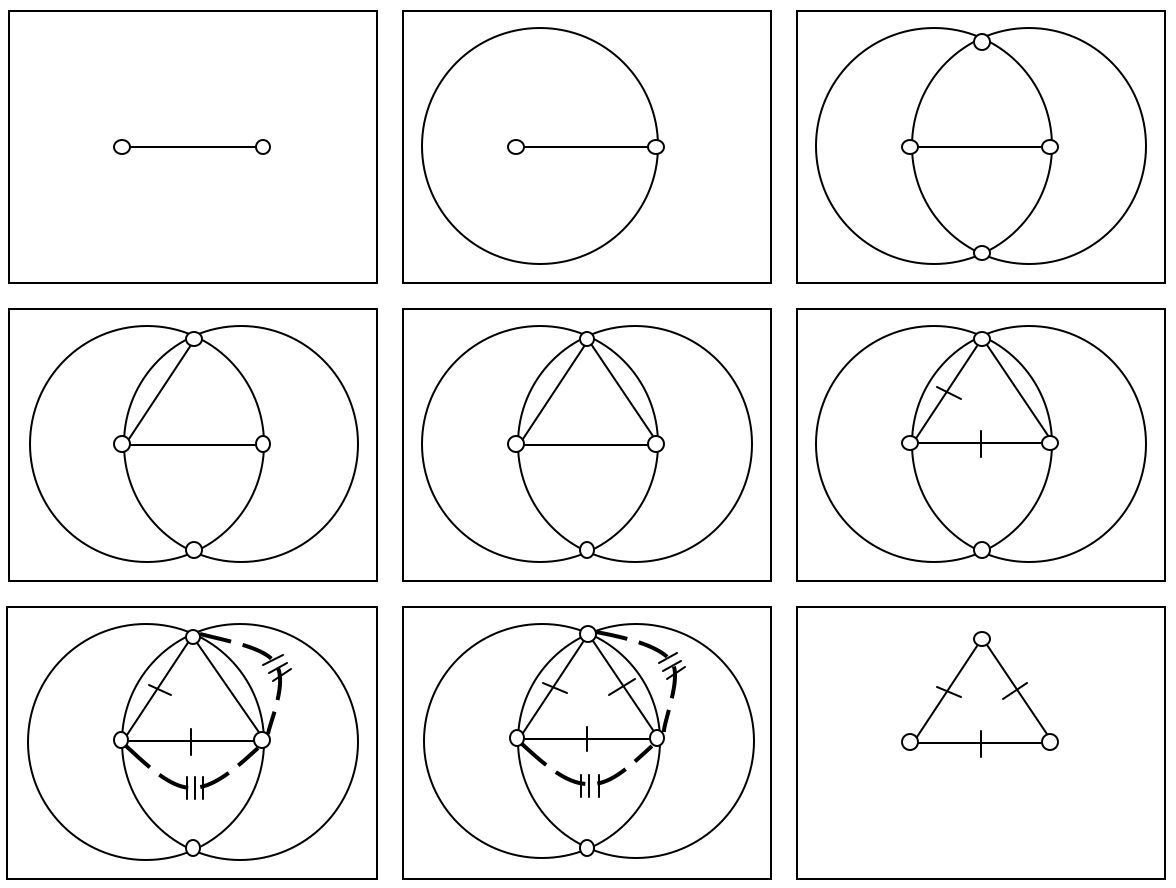Figure 1

What about Tennant’s claim that a proof is “a syntactic object consisting only of sentences” as opposed to diagrams? A proof is never a syntactic object. A formal derivation on its own is a syntactic object but not a proof. Without an interpretation of the language of the formal system the end-formula of the derivation says nothing; and so nothing is proved. Without a demonstration of the system’s soundness with respect to the interpretation, one may lack sufficient reason to believe that all derivable conclusions are true. A formal derivation plus an interpretation and soundness proof can be a proof of the derived conclusion, but that whole package is not a syntactic object. Moreover, the part of the proof which really is a syntactic object, the formal derivation, need not consist solely of sentences; it can consist of diagrams.

With claim (a) disposed of, consider again claim (b) that, while not all diagrammatic thinking in a process of thinking through a proof is superfluous, all non-superfluous diagrammatic thinking will be replaceable by non-diagrammatic thinking in a process of thinking through that same proof. The visual thinking in following the proof of Euclid’s first theorem using Miller’s formal system consists in going through a sequence of diagrams and at each step seeing that the next diagram results from a permitted alteration of the previous diagram. It is clear that in a process that counts as thinking through this proof, the diagrammatic thinking is neither superfluous nor replaceable by non-diagrammatic thinking. That knocks out (b), leaving only (c): some thinking that involves a diagram in thinking through a proof is neither superfluous nor replaceable by non-diagrammatic thinking (without changing the proof).

### 3.2 Visual means in non-formal proving

Mathematical practice almost never proceeds by way of formal systems. Outside the context of formal diagrammatic systems, the use of diagrams is widely felt to be unreliable. A diagram can be unfaithful to the described construction: it may represent something with a property that is ruled out by the description, or without a property that is demanded by the description. This is exemplified by diagrams in the famous argument for the proposition that all triangles are isosceles: the meeting point of an angle bisector and the perpendicular bisector of the opposite side is represented as falling inside the triangle, when it has to be outside (Rouse Ball 1939; Maxwell 1959). Errors of this sort are comparatively rare, usually avoidable with a modicum of care, and not inherent in the nature of diagrams; so they do not warrant a general charge of unreliability.

The major sort of error is unwarranted generalisation. Typically diagrams (and other non-verbal visual representations) do not represent their objects as having a property that is actually ruled out by the intention or specification of the object to be represented. But diagrams very frequently do represent their objects as having properties that, though not ruled out by the specification, are not demanded by it. Verbal descriptions can be discrete, in that they supply no more information than is needed. But visual representations are typically indiscrete, in that they supply too much detail. This is often unavoidable, because for many properties or kinds $$F$$, a visual representation cannot represent something as being $$F$$ without representing it as being $$F$$ in a particular way. Any diagram of a triangle, for instance, must represent it as having three acute angles or as having just two acute angles, even if neither property is required by the specification, as would be the case if the specification were “Let ABC be a triangle”. As a result there is a danger that in using a diagram to reason about an arbitrary instance of class $$K$$, we will unwittingly rely on a feature represented in the diagram that is not common to all instances of the class $$K$$. Thus the risk of unwarranted generalisation is a danger inherent in the use of many diagrams.

Indiscretion of diagrams is not confined to geometrical figures. The dot or pebble diagrams of ancient mathematics used to convince one of elementary truths of number theory necessarily display particular numbers of dots, though the truths are general. Here is an example, used to justify the formula for the $$n$$th triangular number, i.e., the sum of the first $$n$$ positive integers.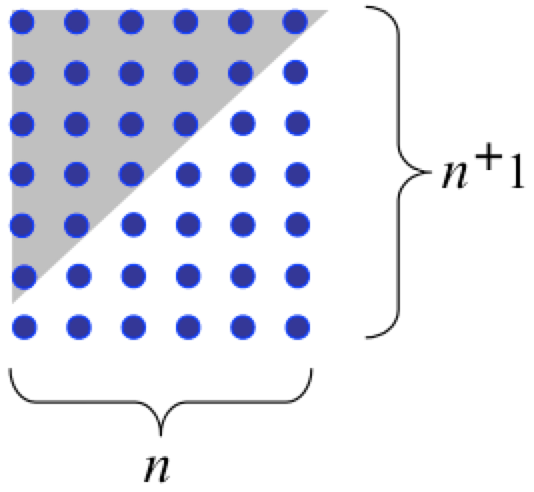Figure 2

The conclusion drawn is that the sum of integers from 1 to $$n$$ is $$(n \times n+1)/2$$ for any positive integer $$n$$, but the diagram presents the case for $$n = 6$$. We can perhaps avoid representing a particular number of dots when we merely imagine a display of the relevant kind; or if a particular number is represented, our experience may not make us aware of the number—just as, when one imagines the sky on a starry night, for no particular number $$k$$ are we aware that exactly $$k$$ stars are represented. Even so, there is likely to be some extra specificity. For example, in imagining an array of dots of the form just illustrated, one is unlikely to imagine just two columns of three dots, the rectangular array for $$n = 2$$. Typically the subject will be aware of imagining an array with more than two columns. This entails that an image is likely to have unintended exclusions. In this case it would exclude the three-by-two array. An image of a triangle representing all angles as acute would exclude triangles with an obtuse angle or a right angle. The danger is that the visual reasoning will not be valid for the cases that are unintentionally excluded by the visual representation, with the result that the step to the conclusion is an unwarranted generalisation.

What should we make of this? First, let us note that in a few cases the image or diagram will not be over-specific. When in geometry all instances of the relevant class are congruent to one another, for instance all circles or all squares, the image or diagram will not be over-specific for a generalisation about that class; so there will be no unintended exclusions and no danger of unwarranted generalisation. Here then are possibilities for reliable visual thinking in proving.

To get clear about the other cases, where there is a danger of over generalizing, it helps to look at generalisation in ordinary non-visual reasoning. Schematically put, in reasoning about things of kind $$K$$, once we have shown that from certain premisses it follows that such-and-such a condition is true of arbitrary instance $$c$$, we can validly infer from those same premisses that that condition is true of all $$K$$s, with the proviso that neither the condition nor any premiss mentions $$c$$. The proviso is required, because if a premiss or the condition does mention $$c$$, the reasoning may depend on a property of $$c$$ that is not shared by all other $$K$$s and so the generalisation would be unsafe. For a trivial example consider a step from “$$x = c$$” to “$$\forall x [x = c]$$”.

A question we face is whether, in order to come to know the truth of a conclusion by following an argument involving generalisation on an arbitrary instance (a.k.a. universal generalisation, or universal quantifier introduction), the thinking must include a conscious, explicit check that the proviso is met. It is clearly not enough that the proviso is in fact met. For in that case it might just be the thinker’s good luck that the proviso is met; hence the thinker would not know that the generalisation is valid and so would not have genuinely thought through the proof at that step.

This leaves two options. The strict option is that without a conscious, explicit check one has not really thought through the proof. The relaxed option is that one can properly think through the proof without checking that the proviso is met, but only if one is sensitive to the potential error and would detect it in otherwise similar arguments. For then one is not just lucky that the proviso is met. Being sensitive in this context consists in being alert to dependence on features of the arbitrary instance not shared by all members of the class of generalisation, a state produced by a combination of past experience and current vigilance. Without compelling reason to prefer one of these options, decisions on what is to count as proving or following a proof must be conditional.

How does all this apply to generalizing from visual thinking about an arbitrary instance? Take the example of the visual route to the formula for triangular numbers using the diagram of Figure 2. The diagram reveals that the formula holds for the 6th triangular number. The generalisation to all triangular numbers is justified only if the visuo-spatial method used is applicable to the $$n$$th triangular number for all positive integers $$n$$, that is, provided that the method used does not depend on a property not shared by all positive integers. A conscious, explicit check that this proviso is met requires making explicit the method exemplified for 6 and proving that the method is applicable for all positive integers in place of 6. (For a similar idea in the context of automating visual arguments, see Jamnik 2001). This is not done in practice when thinking visually, and so if we accept the strict option for thinking through a proof involving generalisation, we would have to accept that the visual route to the formula for triangular numbers does not amount to thinking through a proof of it; and the same would apply to the familiar visual routes to other general positive integer formulas, such as that $$n^2 =$$ the sum of the first $$n$$ odd numbers.

But what if the strict option for proving by generalisation on an arbitrary instance is too strict, and the relaxed option is right? When arriving at the formula in the visual way indicated, one does not pay attention to the fact that the visual display represents the situation for the 6th triangular number; it is as if the mind had somehow extracted a general schema of visual reasoning from exposure to the particular case, and had then proceeded to reason schematically, converting a schematic result into a universal proposition. What is required, on the relaxed option, is sensitivity to the possibility that the schema is not applicable to all positive integers; one must be so alert to ways a schema of the given kind can fall short of universal applicability that if one had been presented with a schema that did fall short, one would have detected the failure.

In the example at hand, the schema of visual reasoning involves at the start taking a number $$k$$ to be represented by a column of $$k$$ dots, thence taking the triangular array of $$n$$ columns to represent the sum of the first $$n$$ positive integers, thence taking that array combined with an inverted copy to make a rectangular array of $$n$$ columns of $$n+1$$ dots. For a schema starting this way to be universally applicable, it must be possible, given any positive integer $$n$$, for the sum of the first $$n$$ positive integers to be represented in the form of a triangular array, so that combined with an inverted copy one gets a rectangular array. This actually fails at the extreme case: $$n = 1$$. The formula $$(n.(n + 1))/2$$ holds for this case; but that is something we know by substituting “1” for the variable in the formula, not by the visual method indicated. That method cannot be applied to $$n = 1$$, because a single dot does not form a triangular array, and combined with a copy it does not form a rectangular array. But we can check that the method works for all positive integers after the first, using visual reasoning to assure ourselves that it works for 2 and that if the method works for $$k$$ it works for $$k+1$$. Together with this reflective thinking, the visual thinking sketched earlier constitutes following a proof of the formula for the $$n$$th triangular number for all integers $$n > 1$$, at least if the relaxed view of thinking through a proof is correct. Similar conclusions hold in the case of other “dot” arguments (Giaquinto 1993, 2007: ch. 8). So in some cases when the visual representation carries unwanted detail, the danger of over-generalisation in visual reasoning can be overcome.

But the fact that this is frequently missed by commentators suggests that the required sensitivity is often absent. Missing an untypical case is a common hazard in attempts at visual proving. A well-known example is the proof of Euler’s formula $$V - E + F = 2$$ for polyhedra by “removing triangles” of a triangulated planar projection of a polyhedron. One is easily convinced by the thinking, but only because the polyhedra we normally think of are convex, while the exceptions are not convex. But it is also easy to miss a case which is not untypical or extreme when thinking visually. An example is Cauchy’s attempted proof (Cauchy 1813) of the claim that if a convex polygon is transformed into another polygon keeping all but one of the sides constant, then if some or all of the internal angles at the vertices increase, the remaining side increases, while if some or all of the internal angles at the vertices decrease, the remaining side decreases. The argument proceeds by considering what happens when one transforms a polygon by increasing (or decreasing) angles, angle by angle. But in a trapezoid, changing a single angle can turn a convex polygon into a concave polygon, and this invalidates the argument (Lyusternik 1963).

The frequency of such mistakes indicates that visual arguments (other than symbol manipulations) often lack the transparency required for proof. Even when a visual argument is in fact sound, its soundness may not be clear, in which case the argument is not a way of proving the truth of the conclusion, though it may be a way of discovering it. But this is consistent with the claim that visual non-symbolic thinking can be (and often is) part of a way of proving something.

An example from knot theory will substantiate the modal part of this claim. To present the example, we need some background information, which will be given with a minimum of technical detail.

A knot is a tame closed non-self-intersecting curve in Euclidean 3-space.

In other words, knots are just the tame curves in Euclidean 3-space which are homeomorphic to a circle. The word “tame” here stands for a property intended to rule out certain pathological cases, such as curves with infinitely nested knotting. There is more than one way of making this mathematically precise, but we have no need for these details. A knot has a specific geometric shape, size and axis-relative position. Now imagine it to be made of flexible yet unbreakable yarn that is stretchable and shrinkable, so that it can be smoothly transformed into other knots without cutting or gluing. Since our interest in a knot is the nature of its knottedness regardless of shape, size or axis-relative position, the real focus of interest is not just the knot but all its possible transforms. A way to think of this is to imagine a knot transforming continuously, so that every possible transform is realized at some time. Then the thing of central interest would be the object that persists over time in varying forms, with knots strictly so called being the things captured in each particular freeze frame. Mathematically, we represent the relevant entity as an equivalence class of knots.

Two knots are equivalent iff one can be smoothly deformed into the other by stretching, shrinking, twisting, flipping, repositioning or in any other way that does not involve cutting, gluing or passing one strand through another.

The relevant kind of deformation forbids eliminating a knotted part by shrinking it down to a point. Again there are mathematically precise definitions of knot-equivalence. Figure 3 gives diagrams of equivalent knots, instances of a trefoil.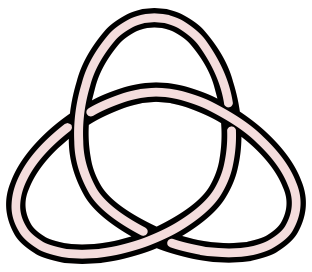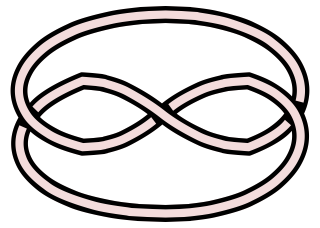(a)
(b)

Figure 3

Diagrams like these are not merely illustrations; they also have an operational role in knot theory. But not any picture of a knot will do for this purpose. We need to specify:

A knot diagram is a regular projection of a knot onto a plane which, when there is a crossing, tells us which strand passes over the other.

Regularity here is a combination of conditions. In particular, regularity entails that not more than two points of the strict knot project to the same point on the plane, and that two points of the strict knot project to the same point on the plane only where there is a crossing. For more on diagrams in knot theory see (De Toffoli and Giardino 2014).

A major task of knot theory is to find ways of telling whether two knot diagrams are diagrams of equivalent knots. In particular we will want to know if a given knot diagram represents a knot equivalent to an unknot, that is, a knot representable by a knot diagram without crossings.

One way of showing that a knot diagram represents a knot equivalent to an unknot is to show that the diagram can be transformed into one without crossings by a sequence of atomic moves, known as Reidemeister moves. The relevant background fact is Reidemeister’s theorem, which links the visualizable diagrammatic changes to the mathematically precise definition of knot equivalence: Two knots are equivalent if and only if there is a finite sequence of Reidemeister moves taking a knot diagram of one to a knot diagram of the other. Figure 4 illustrates. Each knot diagram is changed into the adjacent knot diagram by a Reidemeister move; hence the knot represented by the leftmost diagram is equivalent to the unknot.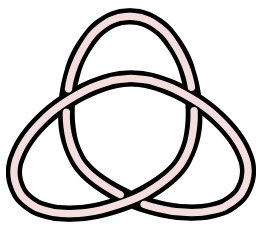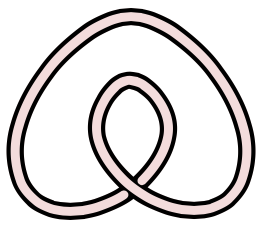(a)
(b)
(c)

Figure 4

In contrast to these, the knot presented by the left knot diagram of Figure 3, a trefoil, may seem impossible to deform into an unknot. And in fact it is. To prove it, we can use a knot invariant known as colourability. An arc in a knot diagram is a maximal part between crossings (or the whole thing if there are no crossings). Colourability is this:

A knot diagram is colourable if and only if each of its arcs can be coloured one of three different colours so that (a) at least two colours are used and (b) at each crossing the three arcs are all coloured the same or all coloured differently.

The reference to colours here is inessential. Colourability is in fact a specific case of a kind of combinatorial property known as mod $$p$$ labelling (for $$p$$ an odd prime). Colourability is a knot invariant in the sense that if one diagram of a knot is colourable every diagram of that knot and of any equivalent knot is colourable. (By Reidemeister’s theorem this can be proved by showing that each Reidemeister move preserves colourability.) A standard diagram of an unknot, a diagram without crossings, is clearly not colourable because it has only one arc (the whole thing) and so two colours cannot be used. So in order to complete proving that the trefoil is not equivalent to an unknot, we only need prove that our trefoil diagram is colourable. This can be done visually. Colour each arc of the knot diagram one of the three colours red, green or blue so that no two arcs have the same colour (or visualize this). Then do a visual check of each crossing, to see that at each crossing the three meeting arcs are all coloured differently. That visual part of the proof is clearly non-superfluous and non-replaceable (without changing the proof). Moreover, the soundness of the argument is quite transparent. So here is a case of a non-formal, non-symbolic visual way of proving a mathematical truth.

### 3.3 A dispute: diagrams in proofs in analysis.

Where notions involving the infinite are in play, such as many involving limits, the use of diagrams is famously risky. For this reason it has been widely thought that, beyond some very simple cases, arguments in real and complex analysis in which diagrams have a non-superfluous role are not genuine proofs. Bolzano  expressed this attitude with regard to the intermediate value theorem for the real numbers (IVT) before giving a purely analytic proof, arguing that spatial thinking could not be used to help justify the IVT. James Robert Brown (1999) takes issue with Bolzano on this point. The IVT is this:

If $$f$$ is a real-valued function of a real variable continuous on the closed interval $$[a, b]$$ and $$f(a) < c < f(b)$$, then for some $$x$$ in $$(a, b), f(x) = c$$.

Brown focuses on the special case when $$c = 0$$. As the IVT can be deduced easily from this special case using the theorem that the difference of two continuous functions is continuous, there is no loss of generality here. Alluding to a diagram like Figure 5, Brown (1999) writes

We have a continuous line running from below to above the $$x$$-axis. Clearly, it must cross that axis in doing so. (1999: 26)

Later he claims:

Using the picture alone, we can be certain of this result—if we can be certain of anything. (1999: 28)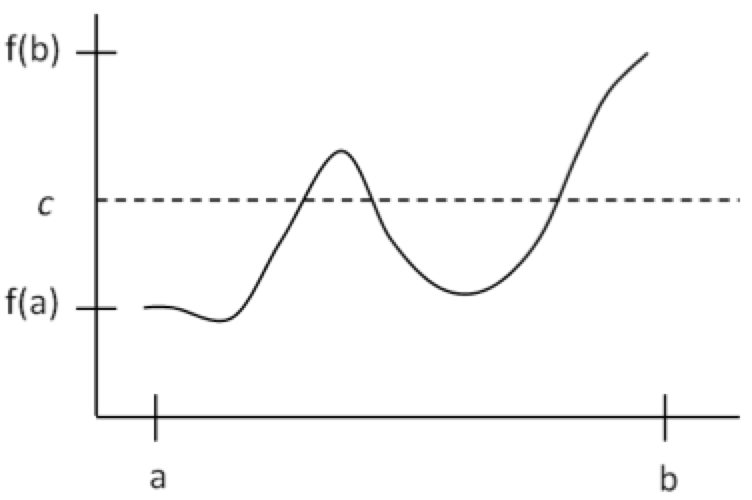Figure 5

Bolzano’s diagram-free proof of the IVT is an argument from what later became known as the Dedekind completeness of the real numbers: every non-empty set of reals bounded above (below) has a least upper bound (greatest lower bound). The value of Bolzano’s deduction of the IVT from the Dedekind completeness of the reals, according to Brown, is not that it proves the IVT but that it gives us confirmation of Dedekind completeness, just as an empirical hypothesis in empirical science gets confirmed by deducing some consequence of the hypothesis and observing those consequence to be true. This view assumes that we already know the IVT to be true by observing a diagram relevantly like Figure 5.

That assumption is challenged by Giaquinto (2011). Once we distinguish graphical concepts from associated analytic concepts, the underlying argument from the diagram is essentially this.

• 1. Any function $$f$$ which is $$\varepsilon\textrm{-}\delta$$ continuous on $$[a, b]$$ with $$f (a) < 0 < f (b)$$ has a visually continuous graphical curve from below the horizontal line representing the $$x$$-axis to above.
• 2. Any visually continuous graphical curve from below a horizontal line to above it meets the line at a crossing point.
• 3. Any function whose graphical curve meets the line representing the $$x$$-axis at a crossing point has a zero value.
• 4. So, any $$\varepsilon\textrm{-}\delta$$ continuous function $$f$$ on $$[a, b]$$ with $$f (a) < 0< f (b)$$ has a zero value.

What is inferred from the diagram is premiss 2. Premisses 1 and 3 are assumptions linking analytical with graphical conditions. These linking assumptions are disputed. With regard to premiss 1 Giaquinto (2011) argues that there are functions on the reals which meet the antecedent condition but do not have graphical curves, such as continuous but nowhere differentiable functions and functions which oscillate with unbounded frequency e.g., $$f(x) = x \cdot\sin(1/x)$$ for non-zero $$x$$ in $$[-1, 1]$$ and $$f(0) = 0$$.

With regard to premiss 3 it is argued that, under the standard conventions of graphical representation of functions in a Cartesian co-ordinate frame, the graphical curve for $$x^2 - 2$$ in the rationals is the same as the graphical curve for $$x^2- 2$$ in the reals. This is because every real is a limit point of rationals; so for every point $$P$$ with one or both co-ordinates irrational, there are points arbitrarily close to $$P$$ with both co-ordinates rational; so no gaps would appear if irrational points were removed from the curve for $$x^2- 2$$ in the reals. But for $$x$$ in the rational interval [0, 2] the function $$x^2- 2$$ has no zero value, even though it has a graphical curve which visually crosses the line representing the $$x$$-axis. So one cannot read off the existence of a zero of $$x^2- 2$$ on the reals from the diagram; one needs to appeal to some property of the reals which the rationals lack, such as Dedekind completeness.

This raises some obvious questions. Do any theorems of analysis have proofs in which diagrams have a non-superfluous role? Littlewood (1953: 54–5) thought so and gives an example which is examined in Giaquinto (1994). If so, can we demarcate this class of theorems by some mathematical feature of their content? Another question is whether there is a significantly broad class of functions on the reals for which we could prove an intermediate value theorem (i.e., restricted to that class).

If there are theorems of analysis provable with diagrams we do not yet have a mathematical demarcation criterion for them. A natural place to look would be O-minimal structures on the reals—this was brought to the author’s attention by Ethan Galebach. This is because of some remarkable theorems about such structures which exclude all the pathological (hence vision-defying) functions on the reals (Van den Dries 1998), such as continuous nowhere differentiable functions and “space-filling” curves i.e., continuous surjections $$f:(0, 1)\rightarrow(0, 1)^2$$. Is the IVT for functions in an O-minimal structure on the reals provable by visual means? Certainly one objection to the visual argument for the unrestricted IVT does not apply when the restriction is in place. This is the objection that continuous nowhere differentiable functions, having no graphical curve, provide counterexamples to the premiss that any $$\varepsilon\textrm{-}\delta$$ continuous function $$f$$ on $$[a, b]$$ with $$f (a) < c < f (b)$$ has a visually continuous graphical curve from below the horizontal line representing $$y = c$$ to above. But the existence of continuous functions with no graphical curve is not the only objection to the visual argument, contrary to a claim of Azzouni (2013: 327). There are also counterexamples to the premiss that any function that does have a graphical curve which visibly crosses the line representing $$y = c$$ takes $$c$$ as a value, e.g., the function $$x^2 - 2$$ on the rationals with $$c = 0$$. So the question of a visual proof of the IVT restricted to functions in an O-minimal structure on the reals is still open at the time of writing.

## 4. Visual thinking and discovery

Though philosophical discussion of visual thinking in mathematics has concentrated on its role in proof, visual thinking may be more valuable for discovery than proof. Three kinds of discovery important in mathematical practice are these:

• (1)propositional discovery (discovering, of a proposition, that it is true),
• (2)discovering a proof strategy (or more loosely, getting the idea for a proof of a proposition), and
• (3)discovering a property or kind of mathematical entity.

In the following subsections visual discovery of these kinds will be discussed and illustrated.

### 4.1 Propositional discovery

To discover a truth, as that expression is being used here, is to come to believe it by one’s own lights (as opposed to reading it or being told) in a way that is reliable and involves no violation of epistemic rationality (given one’s epistemic state). One can discover a truth without being the first to discover it (in this context); it is enough that one comes to believe it in an independent, reliable and rational way. The difference between merely discovering a truth and proving it is a matter of transparency: for proving or following a proof the subject must be aware of the way in which the conclusion is reached and the soundness of that way; this is not required for discovery.

Sometimes one discovers something by means of visual thinking using background knowledge, resulting in a cogent argument from which one could construct a proof. A nice example is a visual argument that any knot diagram with a finite number of crossings can be turned into a diagram of an unknot by interchanging the over-strand and under-strand of some of its crossings (Adams 2001: 58–90). That argument is a bit too long to present accessibly here. For a short example, here is a way of discovering that the geometric mean of two positive numbers is less than or equal to their arithmetic mean (Eddy 1985) using Figure 6.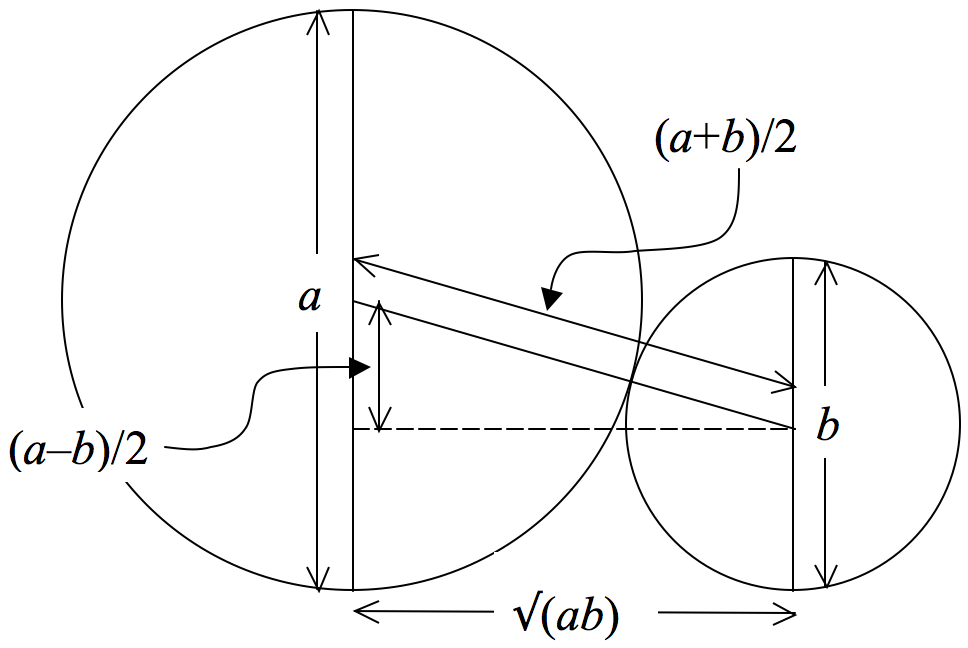Figure 6

Two circles (with diameters $$a$$ and $$b$$) meet at a single point. A line is drawn between their centres through their common point; its length is $$(a + b)/2$$, the sum of the two radii. This line is the hypotenuse of a right angled triangle with one other side of length $$(a - b)/2$$, the difference of the radii. Pythagoras’s theorem is used to infer that the remaining side of the right-angled triangle has length $$\sqrt{(ab)}$$.Then visualizing what happens to the triangle when the diameter of the smaller circle varies between 0 and the diameter of the larger circle, one infers that $$0 < \sqrt{(ab)} < (a + b)/2$$; then verifying symbolically that $$\sqrt{(ab)} = (a + b)/2$$ when $$a = b$$, one concludes that for positive $$a$$ and $$b$$, $$\sqrt{(ab)} \le (a + b)/2$$.

This thinking does not constitute a case of proving or following a proof of the conclusion, because it involves a step which we cannot clearly tell is valid. This is the step of attempting to visually imagine what would happen when the smaller circle varies in diameter between 0 and the diameter of the larger circle and inferring from the resulting experience that the line joining the centres of the circles will always be longer than the horizontal line from the centre of the smaller circle to the vertical diameter of the larger circle. This step seems sound (does not lead us into error) and may be sound; but its soundness is opaque. If in fact it is sound, the whole thinking process is a reliable way of reaching the conclusion; so in the absence of factors that would make it irrational to trust the thinking, it would be a way of discovering the conclusion to be true.

### 4.2 Discovering a proof strategy

In some cases visual thinking inclines one to believe something on the basis of assumptions suggested by the visual representation that remain to be justified given the subject’s current knowledge. In such cases there is always the danger that the subject takes the visual representation to show the correctness of the assumptions and ends up with an unwarranted belief. In such a case, even if the belief is true, the subject has not made a discovery, as the means of belief-acquisition is unreliable. Here is an example using Figure 7 (Montuchi and Page 1988).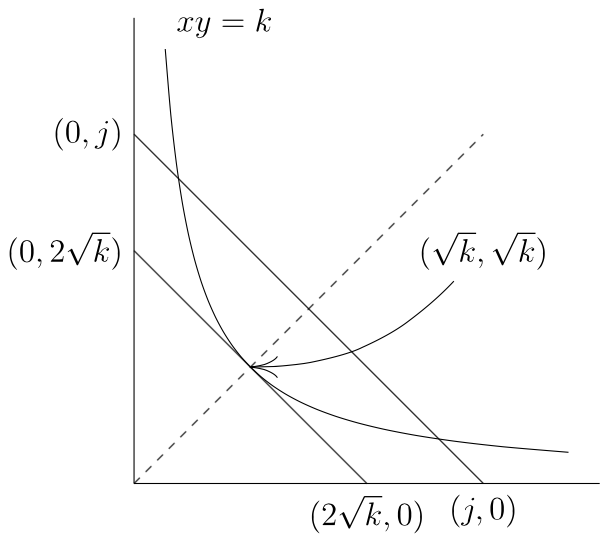Figure 7

Using this diagram one can come to think the following about the real numbers. When for a constant $$k$$ the positive values of $$x$$ and $$y$$ are constrained to satisfy the equation $$x \cdot y = k$$, the positive values of $$x$$ and $$y$$ for which $$x + y$$ is minimal are $$x = \sqrt{k} = y$$. (Let “#” denote this claim.)

Suppose that one knows the conventions for representing functions by graphs in a Cartesian co-ordinate system, knows also that the diagonal represents the function $$y = x$$, and that a line segment with gradient –1 from $$(0, b)$$ to $$(b, 0)$$ represents the function $$x + y = b$$. Then looking at the diagram may incline one to think that for no positive value of $$x$$ does the value of $$y$$ in the function $$x\cdot y = k$$ fall below the value of $$y$$ in $$x + y = 2\sqrt{k}$$, and that these functions coincide just at the diagonal. From these beliefs the subject may (correctly) infer the conclusion #. But mere attention to the diagram cannot warrant believing that, for a given positive $$x$$-value, the $$y$$-value of $$x\cdot y = k$$ never falls below the $$y$$-value of $$x + y = 2\sqrt{k}$$ and that the functions coincide just at the diagonal; for the conventions of representation do not rule out that the curve of $$x\cdot y = k$$ meets the curve of $$x + y = 2\sqrt{k}$$ at two points extremely close to the diagonal, and that the former curve falls under the latter in between those two points. So the visual thinking is not in this case a means of discovering proposition #.

But it is useful because it provides the idea for a proof of the conclusion—one of the major benefits of visual thinking in mathematics. In brief: for each equation $$(x\cdot y = k$$; $$x + y = 2\sqrt{k})$$ if $$x = y$$, their common value is $$\sqrt{k}$$. So the functions expressed by those equations meet at the diagonal. To show that, for a fixed positive $$x$$-value, the $$y$$-values of $$x\cdot y = k$$ never fall below the $$y$$-values of $$x + y = 2\sqrt{k}$$, it suffices to show that $$2\sqrt{k} - x \le k/x$$. As a geometric mean is less than or equal to the corresponding arithmetic mean, $$\sqrt{[x \cdot (k/x)]} \le [x + (k/x)]/2$$. So $$2\sqrt{k} \le x + (k/x)$$. So $$2\sqrt{k} - x \le k/x$$.

In this example, visual attention to, and reasoning about, the diagram is not part of a way of discovering the conclusion. But if it gave one the idea for the argument just given, it would be part of what led to a way of discovering the conclusion, and that is important.

Can visual thinking lead to discovery of an idea for a proof in more advanced contexts? Yes. Carter (2010) gives an example from free probability theory. The case is about certain permutations (those denoted by “$$p$$” with a circumflex in Carter 2010) on a finite set of natural numbers. Using specific kinds of diagram, easily seen properties of the diagrams lead one naturally to certain properties of the permutations (crossing and non-crossing, having neighbouring pairs), and to a certain operation (cancellation of neighbouring pairs). All of these have algebraic definitions, but the ideas defined were noticed by thinking in terms of the diagrams. For the relevant permutations $$\sigma$$, $$\sigma(\sigma(n)) = n$$; so a permutation can be represented by a set of lines joining dots. The permutations represented on the left and right in Figure 8 are non-crossing and crossing respectively, the former with neighbouring pairs $$\{2, 3\}$$ and $$\{6, 7\}$$.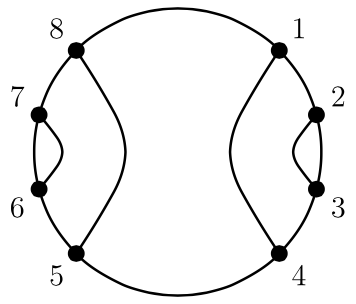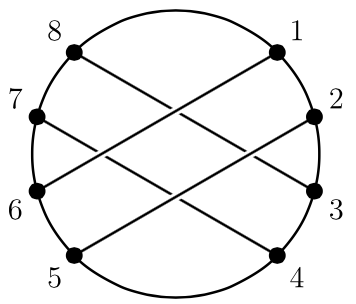(a)
(b)

Figure 8

A permutation $$\sigma$$ of $$\{1, 2, \ldots, 2p\}$$ is defined to have a crossing just when there are $$a$$, $$b$$, $$c$$, $$d$$ in $$\{1, 2, \ldots, 2p\}$$ such that $$a < b < c < d$$ and $$\sigma(a) = c$$ and $$\sigma(b) = d$$. The focus is on the proof of a theorem which employs this notion. (The theorem is that when a permutation of $$\{1, 2, \ldots, 2p\}$$ of the relevant kind is non-crossing, there will be exactly $$p+1$$ R-equivalence classes, where $$R$$ is a certain equivalence relation on $$\{1, 2, \ldots, 2p\}$$ defined in terms of the permutation.) Carter says that the proofs of some lemmas “rely on a visualization of the setup”, in that to grasp the correctness of one or more of the steps one needs to visualize the situation. There is also a nice example of some reasoning in terms of a diagram which gives the idea for a proof (“suggests a proof strategy”) for the lemma that every non-crossing permutation has a neighbouring pair. Reflection on a diagram such as Figure 9 does the work.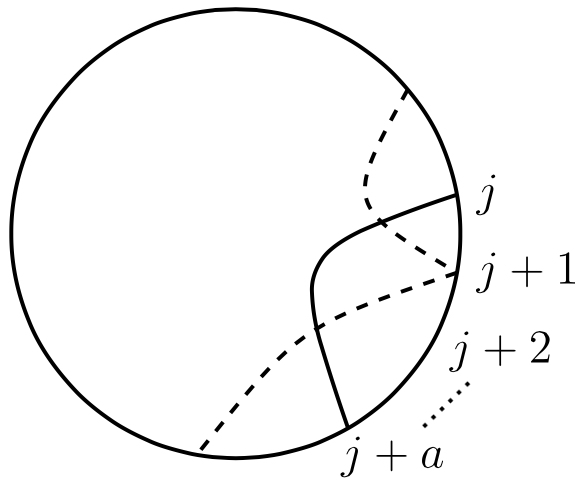Figure 9

The reasoning is this. Suppose that $$\pi$$ has no neighbouring pair. Choose $$j$$ such that $$\pi(j) - j = a$$ is minimal, that is, for all $$k, \pi(j) - j \le \pi(k) - k$$. As $$\pi$$ has no neighbouring pair, $$\pi(j+1) \ne j$$. So either $$\pi(j+1)$$ is less than $$j$$ and we have a crossing, or by minimality of $$\pi(j) - j$$, $$\pi(j+1)$$ is greater than $$j+a$$ and again we have a crossing. Carter reports that this disjunction was initially believed by thinking in term of the diagram, and the proof of the lemma given in the published paper is a non-diagrammatic “version” of that reasoning. In this case study, visual thinking is shown to contribute to discovery in several ways; in particular, by leading the mathematicians to notice crucial properties—the “definitions are based on the diagrams”—and in giving them the ideas for parts of the overall proof.

### 4.3 Discovering properties and kinds

In this section I will illustrate and then discuss the use of visual thinking in discovering kinds of mathematical entity, by going through a few of the main steps leading to geometric group theory, a subject which really took off in the 1980s through the work of Mikhail Gromov. The material is set out nicely in greater depth in Starikova (2012).

Sometimes it can be fruitful to think of non-spatial entities, such as algebraic structures, in terms of a spatial representation. An example is the representation of a finitely generated group by a Cayley graph. Let $$(G, \cdot)$$ be a group and $$S$$ a finite subset of $$G$$. Let $$S^{-1}$$ be the set of inverses of members of $$S$$. Then $$(G, \cdot)$$ is generated by $$S$$ if and only if every member of $$G$$ is the product (with respect to $$\cdot$$) of members of $$S\cup S^{-1}$$. In that case $$(G, \cdot, S)$$ is said to be a finitely generated group. Here are a couple of examples.

First consider the group $$S_{3}$$ of permutations of 3 elements under composition. Letting $$\{a, b, c\}$$ be the elements, all six permutations can be generated by $$\rf$$ and $$\rr$$ where

$$\rf$$ (for “flip”) fixes a and swaps $$b$$ with $$c$$, i.e., it takes to $$\langle a, b, c\rangle$$ to $$\langle a, c, b\rangle$$, and

$$\rr$$ (for “rotate”) takes $$\langle a, b, c\rangle$$ to $$\langle c, a, b\rangle$$.

The Cayley graph for $$(S_{3}, \cdot, \{\rf, \rr\})$$ is a graph whose vertices represent the members of $$S_{3}$$ and two “colours” of directed edges, representing composition with $$\rf$$ and composition with $$\rr$$. Figure 10 illustrates: red directed edges represent composition with $$\rr$$ and black edges represent composition with $$\rf$$. So a red edge from a vertex $$\rv$$ representing $$\rs$$ in $$S_{3}$$ ends at a vertex representing $$\rs\rr$$ and a black edge from $$\rv$$ ends at a vertex representing $$\rs\rf$$. (Notation: “$$\rs\rr$$” abbreviates “$$\rs \cdot \rr$$” which here denotes “$$\rs$$ followed by $$\rr$$”; same for “$$\rf$$” in place of “$$\rr$$”.) A black edge has arrowheads both ways because $$\rf$$ is its own inverse, that is, flipping and flipping again takes you back to where you started. (Sometimes a pair of edges with arrows in opposite directions is used instead.) The symbol “$$\re$$” denotes the identity.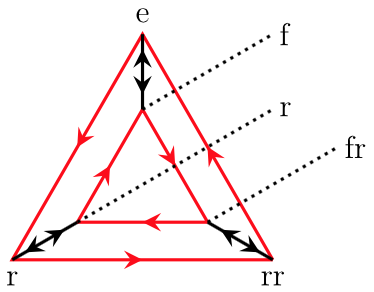Figure 10

An example of a finitely generated group of infinite order is $$(\mathbb{Z}, +, \{1\})$$. We can get any integer by successively adding 1 or its additive inverse $$-1$$. Since 3 added to the inverse of 2 is 1, and 2 added to the inverse of 3 is $$-1$$, we can get any integer by adding members of $$\{2, 3\}$$ and their inverses. Thus both $$\{1\}$$ and $$\{2, 3\}$$ are generating sets for $$(\mathbb{Z}, +)$$. Figure 11 illustrates part of the Cayley graph for $$(\mathbb{Z}, +, \{2, 3\})$$. The horizontal directed edges represent +2. The directed edges ascending or descending obliquely represent $$+3$$.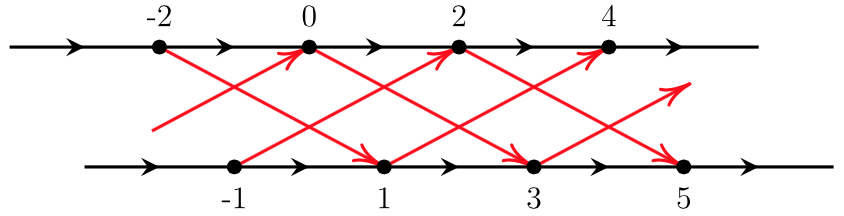Figure 11

Another example of a generated group of infinite order is $$F_2$$, the free group generated by a pair of members. The first few iterations of its Cayley graph are shown in Figure 12, where $$\{a, b\}$$ is the set of generators and a right horizontal move between adjacent vertices represents composition with $$a$$, an upward vertical move represents composition with $$b$$, and leftward and downward moves represent composition with the inverse of $$a$$ and the inverse of $$b$$ respectively. The central vertex represents the identity.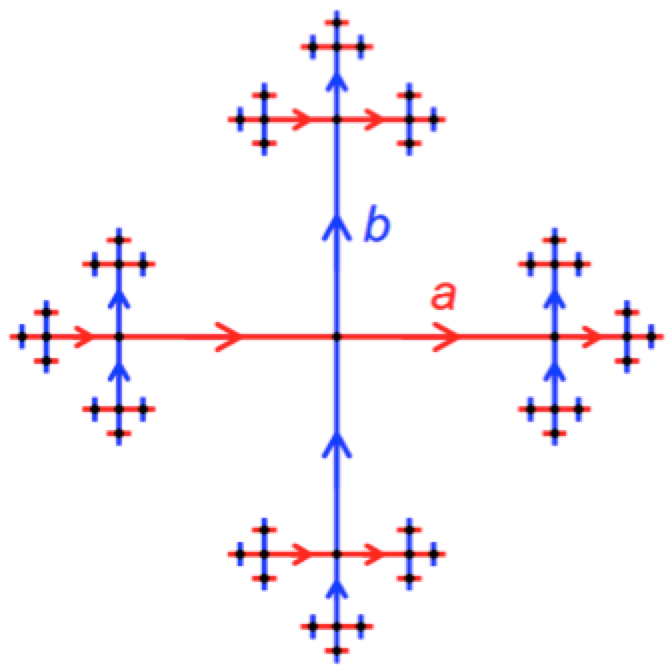Figure 12

Thinking of generated groups in terms of their Cayley graphs makes it very natural to view them as metric spaces. A path is a sequence of consecutively adjacent edges, regardless of direction. For example in the Cayley graph for $$(\mathbb{Z}, +, \{2, 3\})$$ the edges from $$-2$$ to 1, from 1 to $$-1$$, from $$-1$$ to 2 (in that order) constitute a path, representing the action, starting from $$-2$$, of adding 3, then adding $$-2$$, then adding 3. Taking each edge to have unit length, the metric $$d_S$$ for a group $$G$$ generated by a finite subset $$S$$ of $$G$$ is defined: for any $$g$$, $$h \in G$$, $$d_{S}(g, h) =$$ the length of a shortest path from $$g$$ to $$h$$ in the Caley graph of $$(G, \cdot, S)$$. This is the word metric for this generated group.

Viewing a finitely generated group as a metric space allows us to consider its growth function $$\gamma(n)$$ which is the cardinality of the “ball” of radius $$\le n$$ centred on the identity (the number of members of the group whose distance from the identity is not greater than $$n$$). A growth function for a given group depends on the set of generators chosen, but when the group is infinite the asymptotic behaviour as $$n \rightarrow \infty$$ of the growth functions is independent of the set of generators.

Noticing the possibility of defining a metric on generated groups did not require first viewing diagrams of their Cayley graphs. This is because a word in the generators is just a finite sequence of symbols for the generators or their inverses (we omit the symbol for the group operation), and so has an obvious length visually suggested by the written form of the word, namely the number of symbols in the sequence; and then it is natural to define the distance between group members $$g$$ and $$h$$ to be the length of a shortest word that gets one from $$g$$ to $$h$$ by right multiplication, that is, $$\textrm{min}\{\textrm{length}(w): w = g^{-1}h\}$$.

However, viewing generated groups by means of their Cayley graphs was the necessary starting point for geometric group theory, which enables us to view finitely generated groups of infinite order not merely as graphs or metric spaces but as geometric entities. The main steps on this route will be sketched briefly here; for more detail see Starikova (2012) and the references therein. The visual key is to start thinking in terms of the “coarse geometry” of the Cayley graph of the generated group, by zooming out in visual imagination so far that the discrete nature of the graph is transformed into a traditional geometrical object. For example, the Cayley graph of a generated group of finite order such as $$(S_{3}, \cdot, \{f, r\})$$ illustrated in Figure 11 becomes a dot; the Cayley graph for $$(\mathbb{Z}, +, \{2, 3\})$$ illustrated in Figure 12 becomes an uninterrupted line infinite in both directions.

The word metric of a generated group is discrete: the values are always in $$N$$. How is this visuo-spatial association of a discrete metric space with a continuous geometrical object achieved mathematically? By quasi-isometry. While an isometry from one metric space to another is a distance preserving map, a quasi-isometry is a map which preserves distances to within fixed linear bounds. Precisely put, a map $$f$$ from $$(S, d)$$ to $$(S', d')$$ is a quasi-isometry iff for some real constants $$L > 0$$ and $$K \ge 0$$ and all $$x$$, $$y$$ in $$S$$ $d(x, y)/L - K \le d'(f(x), f(y)) \le L \cdot d(x, y) + K.$

The spaces $$(S, d)$$ and $$(S', d')$$ are quasi-isometric spaces iff the quasi-isometry $$f$$ is also quasi-surjective, in the sense that there is a real constant $$M \ge 0$$ such that every point of $$S'$$ is no further than $$M$$ away from some point in the image of $$f$$.

For example, $$(\mathbb{Z}, d)$$ is quasi-isometric to $$(\mathbb{R}, d)$$ where $$d(x, y) = |y - x|$$, because the inclusion map $$\iota$$ from $$\mathbb{Z}$$ to $$\mathbb{R}$$, $$\iota(n) = n$$, is an isometry hence a quasi-isometry with $$L = 1$$ and $$K = 0$$, and each point in $$\mathbb{R}$$ is no further than $$1/2$$ away from an integer (in $$\mathbb{R}$$). Also, it is easy to see that for any real number $$x$$, if $$g(x) =$$ the nearest integer to $$x$$ (or the greatest integer less than $$x$$ if it is midway between integers) then $$g$$ is a quasi-isometry from $$\mathbb{R}$$ to $$\mathbb{Z}$$ with $$L = 1$$ and $$K =\frac{1}{2}$$;.

The relation between metric spaces of being quasi-isometric is an equivalence relation. Also, if $$S$$ and $$T$$ are generating sets of a group $$(G, \cdot)$$, the Cayley graphs of $$(G, \cdot, S)$$ and $$(G, \cdot, T)$$ with their word metrics are quasi-isometric spaces. This means that properties of a generated group which are quasi-isometric invariants will be independent of the choice of generating set, and therefore informative about the group itself.

Moreover, it is easy to show that the Cayley graph of a generated group with word metric is quasi-isometric to a geodesic space. A triangle with vertices $$x$$, $$y$$, $$z$$ in this space is the union of three geodesic segments, between $$x$$ and $$y$$, between $$y$$ and $$z$$, and between $$z$$ and $$x$$. This is the gateway for the application of Gromov’s insights, some of which can be grasped with the help of visual geometric thinking.

Here are some indications. Recall the Poincaré open disc model of hyperbolic geometry: geodesics are diameters or arcs of circles orthogonal to the boundary, with unit distance represented by ever shorter Euclidean distances as one moves from the centre towards the boundary. (The boundary is not part of the model). All triangles have angle sum $$< \pi$$ (Figure 13, left), and there is a global constant δ such that all triangles are δ-thin in the following sense:

A triangle $$T$$ is δ-thin if and only if any point on one side of $$T$$ lies within δ of some point on one of the other two sides.

This condition is equivalent to the condition that each side of $$T$$ lies within the union of the δ-neighbourhoods of the other two sides, as illustrated in Figure 13, right. There is no constant δ such that all triangles in a Euclidean plane are δ-thin, because for any δ there are triangles large enough that the midpoint of a longest side lies further than δ from all points on the other two sides.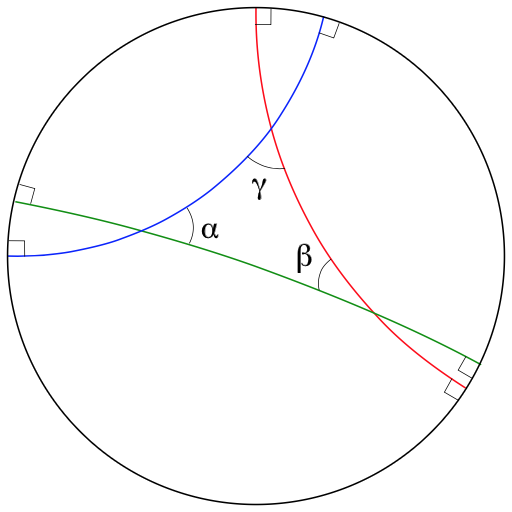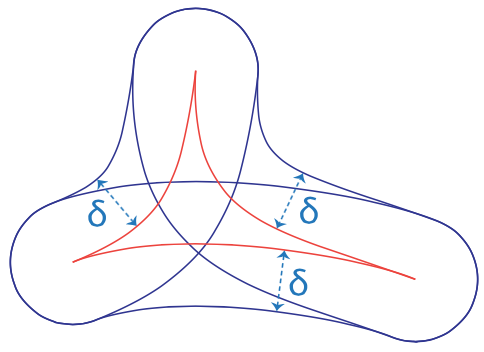(a)
(b)

Figure 13

The definition of thin triangles is sufficiently general to apply to any geodesic space and allows a generalisation of the concept of hyperbolicity beyond its original context:

• A geodesic space is hyperbolic iff for some δ all its triangles are δ-thin.
• A group is hyperbolic iff it has a Cayley graph quasi-isometric to a hyperbolic geodesic space.

The class of hyperbolic groups is large and includes important subkinds, such as finite groups, free groups and the fundamental groups of surfaces of genus $$\ge 2$$. Some striking theorems have been proved for them. For example, for every hyperbolic group the word problem is solvable, and every hyperbolic group has a finite presentation. So we can reasonably conclude that the discovery of this mathematical kind, the hyperbolic groups, has been fruitful.

How important was visual thinking to the discoveries leading to geometric group theory? Visual thinking was needed to discover Cayley graphs as a means of representing finitely generated groups. This is not the triviality it might seem: Cayley graphs must be distinguished from the diagrams we use to present them visually. A Cayley graph is a mathematical representation of a generated group, not a visual representation. It consists of the following components: a set $$V$$ (“vertices”), a set $$E$$ of ordered pairs of members of $$V$$ (“directed edges”) and a partition of $$E$$ into distinguished subsets, (“colours”, each one for representing right multiplication by a particular generator). The Cayley graph of a generated group of infinite order cannot be fully represented by a diagram given the usual conventions of representation for diagrams of graphs, and distinct diagrams may visually represent the same Cayley graph: both diagrams in Figure 14 can be labelled so that under the usual conventions they represent the Cayley graph of $$(S_{3}, \cdot, \{f, r\})$$, already illustrated by Figure 10. So the Cayley graph cannot be a diagram.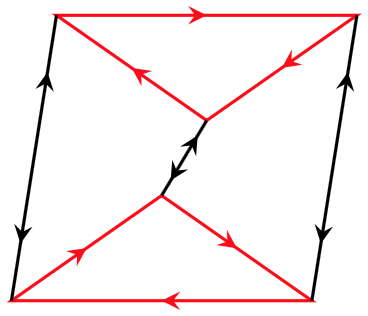(a)
(b)

Figure 14

Diagrams of Cayley graphs were important in prompting mathematicians to think in terms of the coarse-grained geometry of the graphs, in that this idea arises just when one thinks in terms of “zooming out” visually. Gromov (1993) makes the point in a passage quoted in Starikova (2012:138)

This space [a Cayley graph with the word metric] may appear boring and uneventful to a geometer’s eye since it is discrete and the traditional (e.g., topological and infinitesimal) machinery does not run in [the group] Γ. To regain the geometric perspective one has to change one’s position and move the observation point far away from Γ. Then the metric in Γ seen from the distance $$d$$ becomes the original distance divided by $$d$$ and for $$d \rightarrow \infty$$ the points in Γ coalesce into a connected continuous solid unity which occupies the visual horizon without any gaps and holes and fills our geometer’s heart with joy.

In saying that one has to move the observation point far away from Γ so that the points coalesce into a unity which occupies the visual horizon, he makes clear that visual imagination is involved in a crucial step on the road to geometric group theory. Visual thinking is again involved in discovering hyperbolicity as a property of general geodesic spaces from thinking about the Poincaré disk model of hyperbolic geometry. It is hard to see how this property would have been discovered without the use of visual resources.

## 5. Visual thinking and mental arithmetic

While there is no reason to think that mental arithmetic (mental calculation in the integers and rational numbers) typically involves much visual thinking, there is strong evidence of substantial visual processing in the mental arithmetic of highly trained abacus users.

In earlier times an abacus would be a rectangular board or table surface marked with lines or grooves along which pebbles or counters could be moved. The oldest surviving abacus, the Salamis abacus, dated around 300 BCE, is a white marble slab, with markings designed for monetary calculation (Fernandes 2015, Other Internet Resources). These were superseded by rectangular frames within which wires or rods parallel to the short sides are fixed, with moveable holed beads on them. There are several kinds of modern abacus — the Chinese suanpan, the Russian schoty and the Japanese soroban for example — each kind with variations. Evidence for visual processing in mental arithmetic comes from studies with well trained users of the soroban, an example of which is shown in Figure 15.Figure 15

Each column of beads represents a power of 10, increasing to the left. The horizontal bar, sometimes called the reckoning bar, separates the beads on each column into one bead of value 5 above and four beads of value 1 below. The number represented in a column is determined by the beads which are not separated from the reckoning bar. A column on which all beads are separated by a gap from the bar represents zero. For example, the number 6059 is represented on a portion of a schematic soroban in Figure 16.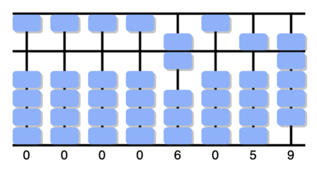Figure 16

On some sorobans there is a mark on the reckoning bar at every third column; if a user chooses one of these as a unit column, the marks will help the user keep track of which columns represent which powers of ten. Calculations are made by using forefinger and thumb to move beads according to procedures for the standard four numerical operations and for extraction of square and cube roots (Bernazzani 2005,Other Internet Resources). Despite the fact that the soroban has a decimal place representation of numbers, the soroban procedures are not ‘translations’ of the procedures normally taught for the standard operations using arabic numerals. For example, multidigit addition on a soroban starts by adding highest powers of ten and proceeds rightwards to lower powers, instead of starting with units thence proceeding leftwards to tens, hundreds and so on.

People trained to use a soroban often learn to do mental arithmetic by visualizing an abacus and imagining moving beads on it in accordance with the procedures learned for arithmetical calculations (Frank and Barner 2012). Mental abacus (MA), as this kind of mental arithmetic is known, compares favourably with other kinds of mental calculation for speed and accuracy (Kojima 1954) and MA users are often found among the medallists in the Mental Calculation World Cup.

Although visual and manual motor imagery is likely to occur, cognitive scientists have probed the question whether the actual processes of MA calculation consist in or involve imagining performing operations on a physical abacus. Brain imaging studies provide one source of evidence bearing on this question. Comparing well-trained abacus calculators with matched controls, evidence has been found that MA involves neural resources of visuospatial working memory with a form of abacus which does not depend on the modality (visual or auditory) of the numerical inputs (Chen et al. 2006). Another imaging study found that, compared to controls without abacus training, subjects with long term MA training from a young age had enhanced brain white matter related to motor and visuospatial processes (Hu et al. 2011).

Behavioural studies provide more evidence. Tests on expert and intermediate level abacus users strongly suggest that MA calculators mentally manipulate an abacus representation so that it passes through the same states that an actual abacus would pass through in solving an addition problem. Without using an actual abacus MA calculators were able to answer correctly questions about intermediates states unique to the abacus-based solution of a problem; moreover, their response times were a monotonic function of the position of the probed state in the sequence of states of the abacus process for solving the problem (Stigler 1984). On top of the ‘intermediate states’ evidence, there is ‘error type’ evidence. Mental addition tests comparing abacus users with American subjects revealed that abacus users made errors of a kind which the Americans did not make, but which were predictable from the distribution of errors in physical abacus addition (Stigler 1984).

Another study found evidence that when a sequence of numbers is presented auditorily (as a verbal whole “three thousand five hundred and forty seven” or as a digit sequence “Three, five, four, seven”) abacus experts encode it into an imaged abacus display, while non-experts encode it verbally (Hishitani 1990).

Further evidence comes from behavioural interference studies. In these studies subjects have to perform mental calculations, with and without a task of some other kind to be performed during the calculation, with the aim of seeing which kinds of task interfere with calculation as measured by differences of reaction time and error rate. An early study found that a linguistic task interfered weakly with MA performance (unless the linguistic task was to answer a mathematical question), while motor and visual tasks interfered relatively strongly. These findings suggested to the paper’s authors that MA representations are not linguistic in nature but rely on visual mechanisms and, for intermediate practitioners, on motor mechanisms as well (Hatano et al. 1977).

These studies provide impressive evidence that MA does involve mental manipulation of a visualized abacus. However, limits of the known capacities for perceiving or representing pluralities of objects seem to pose a problem. We have a parallel individuation system for keeping track of up to four objects simultaneously and an approximate number system (ANS) which allows us to gauge roughly the cardinality of a set of things, with an error which increases with the size of the set. The parallel individuation system has a limit of three or four objects and the ANS represents cardinalities greater than four only approximately. Yet mental abacus users would need to hold in mind with precision abacus representations involving a much larger number of beads than four (and the way in which those beads are distributed on the abacus). For example, the number 439 requires a precise distribution of twelve beads. Frank and Barner (2012) address this problem. In some circumstances we can perceive a plurality of objects as a single entity, a set, and simultaneously perceive those objects as individuals. There is evidence that we can keep track of up to three such sets in parallel and simultaneously make reliable estimates of the cardinalities of the sets (if not more than four). If the sets themselves can be easily perceived as (a) divided into disjoint subsets, e.g. columns of beads on an abacus, and (b) structured in a familiar way, e.g. as a distribution of four beads below a reckoning bar and one above, we have the resources for recognising a three-digit number from its abacus representation. The findings of (Frank and Barner 2012) suggest that this is what happens in MA: a mental abacus is represented in visuospatial working memory by splitting it into a series of columns each of which is stored as a unit with its own detailed substructure.

These cognitive investigations confirm the self-reports of mental abacus users that they calculate mentally by visualizing operating on an abacus as they would operate on a physical abacus. (See the 20-second movie Brief interview with mental abacus user, at the Stanford Language and Cognition Lab, for one such self-report.) There is good evidence that MA often involves processes linked to motor cognition in addition to active visual imagination. Intermediate abacus users often make hand movements, without necessarily attending to those movements during MA calculation, as shown in the second of the three short movies just mentioned. Experiments to test the possible role of motor processes in MA resulted in findings which led the authors to conclude that premotor processes involved in the planning of hand movements were involved in MA (Brooks et al. 2018).

## 6. A priori and a posteriori roles of visual experience

In coming to know a mathematical truth visual experience can play a merely “enabling” role. For example, visual experience may have been a factor in a person’s getting certain concepts involved in a mathematical proposition, thus enabling her to understand the proposition, without giving her reason to believe it. Or the visual experience of reading an argument in a text book may enable one to find out just what the argument is, without helping her tell that the argument is sound. In earlier sections visual experience has been presented as having roles in proof and propositional discovery that are not merely enabling. On the face of it this raises a puzzle: mathematics, as opposed to its application to natural phenomena, has traditionally been thought to be an a priori science; but if visual experience plays a role in acquiring mathematical knowledge which is not merely enabling, the result would surely be a posteriori knowledge, not a priori knowledge. Setting aside knowledge acquired by testimony (reading or hearing that such-&-such is the case), there remain plenty of cases where sensory experience seems to play an evidential role in coming to know some mathematical fact.

### 6.1 Evidential uses of visual experience

A plausible example of the evidential use of sensory experience is the case of a child coming to know that $$5 + 3 = 8$$ by counting on her fingers. While there may be an important $$a$$ priori element in the child’s appreciation that she can reliably generalise from the result of her counting experiment, getting that result by counting is an a posteriori route to it. For another example, consider the question: how many vertices does a cube have? With the background knowledge that cubes do not vary in shape and that material cubes do not differ from geometrical cubes in number of vertices (where a “vertex” of a material cube is a corner), one can find the answer by visually inspecting a material cube. Or if one does not have a material cube to hand, one can visually imagine a cube, and by attending to its top and bottom faces extract the information that the vertices of the cube are exactly the vertices of these two quadrangular faces. When one gets the answer by inspecting a material cube, the visual experience contributes to one’s grounds for believing the answer and that contribution is part of what makes the belief state knowledge. So the role of the visual experience is evidential; hence the resulting knowledge is not a priori. When one gets the answer by visually imagining a cube, one is drawing on the accumulated cognitive effects of past experiences of seeing material cubes to bring to mind what a cube looks like; so the experience of visual imagining has an indirectly evidential role in this case.

Do such examples show that mathematics is not an a priori science? Yes, if an a priori science is understood to be one whose knowable truths are all knowable only in an a priori way, without use of sense experience as evidence. No, if an a priori science is one whose knowable truths are all knowable in an a priori way, allowing that some may be knowable also in an a posteriori way.

### 6.2 An evidential use of visual experience in proving

Many cases of proving something (or following a proof of it) involve making, or imagining making, changes in a symbol array. A standard presentation of the proof of left-cancellation in group theory provides an example. “Left-cancellation” is the claim that for any members $$a$$, $$b$$, $$c$$ of a group with operation $$\cdot$$ and identity element $$\mathbf{e}$$, if $$a \cdot b = a \cdot c$$, then $$b = c$$. Here is (the core of) a proof of it:

\begin{align*} a \cdot b &= a \cdot c\\ a^{-1} \cdot (a \cdot b) &= a^{-1} \cdot (a \cdot c)\\ (a^{-1} \cdot a) \cdot b &= (a^{-1} \cdot a) \cdot c\\ \mathbf{e}\cdot b &= \mathbf{e} \cdot c\\ b &= c. \end{align*}

Suppose that one comes to know left-cancellation by following this sequence of steps. Is this an a priori way of getting this knowledge? Although following a mathematical proof is thought to be a paradigmatically a priori way of getting knowledge, attention to the role of visual experience here throws this into doubt. The case for claiming that the visual experience has an evidential role is as follows.

The visual experience reveals not only what the steps of the argument are but also that they are valid, thereby contributing to our grounds for accepting the argument and believing its conclusion. Consider, for example, the step from the second equation to the third. The relevant background knowledge, apart from the logic of identity, is that a group operation is associative. This fact is usually represented in the form of an equation that simply relocates brackets in an obvious way:

$x \cdot (y \cdot z) = (x \cdot y) \cdot z$

We see that relocating the brackets in accord with this format, the left-hand term of the second equation is transformed into the left-hand term of the third equation, and the same for the right-hand terms. So the visual experience plays an evidential role in our recognising as valid the step from the second equation to the third. Hence this quite standard route to knowledge of left-cancellation turns out to be a posteriori, even though it is a clear case of following a proof.

Against this, one may argue that the description just given of what is going on in following the proof is not strictly correct, as follows. Exactly the same proof can be expressed in natural language, using “the composition of $$x$$ with $$y$$” for “$$x \cdot y$$”, but the result would be hard to take in. Or the proof can be presented using a different notational convention, one which forces a quite different expression of associativity. For example, we can use the Polish convention of putting the operation symbol before the operands: instead of “$$x \cdot y$$” we put “$$\cdot x y$$”. In that case associativity would be expressed in the following way, without brackets:

$\cdot x \cdot y z = \cdot \cdot x y z.$

The equations of the proof would then need to be re-symbolised; but what is expressed by each equation after re-symbolisation and the steps from one to the next would be exactly as before. So we would be following the very same proof, step by step. But we would not be using visual experiences involved to notice the relocation of brackets this time. This suggests that the role of the different visual experiences involved in following the argument in its different guises is merely to give us access to the common reasoning: the role of the experience is merely enabling. On this account the visual experience does not strictly and literally enable us to see that any of the steps are valid; rather, recognition of (or sensitivity to) the validity of the steps results from cognitive processing at a more abstract level.

Which of these rival views is correct? Does our visual experience in following the argument presented with brackets (1) reveal to us the validity of some of the steps, given the relevant background knowledge ? Or (2) merely give us access to the argument? The core of the argument against view (1) is this:

Seeing the relocation of brackets is not essential to following the argument.

So seeing merely gives access to the argument; it does not reveal any step to be valid.

The step to this conclusion is faulty. How one follows a proof may, and in this case does, depend on how it is presented, and different ways of following a proof may be different ways of coming to know its conclusion. While seeing the relocation of brackets is not essential to all ways of following this argument, it is essential to the normal way of following the argument when it is symbolically presented with brackets in the way given above.

Associativity, expressed without symbols, is this: When the binary group operation is applied twice in succession on an ordered triple of operands $$\langle a, b, c\rangle$$, it makes no difference whether the first application is to the initial two operands or the final two operands. While this is the content of associativity, for ease of processing associativity is almost always expressed as a symbol-manipulation rule. Visual perception is used to tell in particular cases whether the rule thus expressed is correctly implemented, in the context of prior knowledge that the rule is correct. What is going on here is a familiar division of labour in mathematical thinking. We first establish the soundness of a rule of symbol-manipulation (in terms of the governing semantic conventions—in this case the matter is trivial); then we check visually that the rule is correctly implemented. Processing at a more abstract, semantic level is often harder than processing at a purely syntactic level; it is for this reason that we often resort to symbol-manipulation techniques as proxy for reasoning directly with meanings to solve a problem. (What is six eighths divided by three fifths, without using any symbolic technique?) When we do use symbol-manipulation in proving or following a proof, visual experience is required to discern that the moves conform to permitted patterns and thus contributes to our grounds for accepting the argument. Then the way of coming to know the conclusion has an a posteriori element.

### 6.3 A non-evidential use of visual experience

Must a use of visual experience in knowledge acquisition be evidential, if the visual experience is not merely enabling? Here is an example which supports a negative answer. Imagine a square or look at a drawing of one. Each of its four sides has a midpoint. Now visualize the “inner” square whose sides run between the midpoints of adjacent sides of the original square (Figure 17, left). By visualizing this figure, it should be clear that the original square is composed precisely of the inner square plus four corner triangles, each side of the inner square being the base of a corner triangle. One can now visualize the corner triangles folding over, with creases along the sides of the inner square. The starting and end states of the imagery transformation can be represented by the left and right diagrams of Figure 17.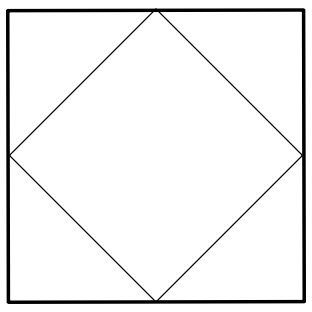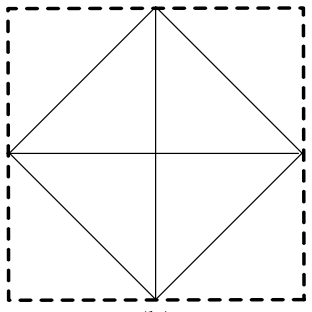(a)
(b)

Figure 17

Visualizing the folding-over within the remembered frame of the original square results in an image of the original square divided into square quarters, its quadrants, and the sides of the inner square seem to be diagonals of the quadrants. Many people conclude that the corner triangles can be arranged to cover the inner square exactly, without any gap or overlap. Thence they infer that the area of the original square is twice the size of the inner square. Let us assume that the propositions concerned are about Euclidean figures. Our concern is with the visual route to the following:

The parts of a square beyond its inner square (formed by joining midpoints of adjacent sides of the original square) can be arranged to fit the inner square exactly, without overlap or gap, without change of size or shape.

The experience of visualizing the corner triangles folding over can lead one to this belief. But it cannot provide good evidence for it. This is because visual experience (of sight or imagination) has limited acuity and so does not enable us to discriminate between a situation in which the outer triangles fit the inner square exactly and a situation in which they fit inexactly but well enough for the mismatch to escape visual detection. (This contrasts with the case of discovering the number of vertices of a cube by seeing or visualizing one.) Even though visualizing the square, the inner square and then visualizing the corner triangles folding over is constrained by the results of earlier perceptual experience of scenes with relevant similarities, we cannot draw from it reliable information about exact equality of areas, because perception itself is not reliable about exact equalities (or exact proportions) of continuous magnitudes.

Though the visual experience could not provide good evidence for the belief, it is possible that we erroneously use the experience evidentially in reaching the belief. But it is also possible, when reaching the belief in the way described, that we do not take the experience to provide evidence. A non-evidential use is more likely, if when one arrives at the belief in this way one feels fairly certain of it, while aware that visual perception and imagination have limited acuity and so cannot provide evidence for a claim of exact fit.

But what could the role of the visualizing experience possibly be, if it were neither merely enabling nor evidential? One suggestion is that we already have relevant beliefs and belief-forming dispositions, and the visualizing experience could serve to bring to mind the beliefs and to activate the belief-forming dispositions (Giaquinto 2007). These beliefs and dispositions will have resulted from prior possession of cognitive resources, some subject-specific such as concepts of geometrical figures, some subject-general such as symmetry perception about perceptually salient vertical and horizontal axes. A relevant prior belief in this case might be that a square is symmetric about a diagonal. A relevant disposition might be the disposition to believe that the quadrants of a square are congruent squares upon seeing or visualizing a square with a horizontal base plus the vertical and horizontal line segments joining midpoints of its opposite sides. (These dispositions differ from ordinary perceptual dispositions to believe what we see in that they are not cancelled when we mistrust the accuracy of the visual experience.)

The question whether the resulting belief would be knowledge depends on whether the belief-forming dispositions are reliable (truth-conducive) and the pre-existing belief states are states of knowledge. As these conditions can be met without any violation of epistemic rationality, the visualizing route described incompletely here can be a route to knowledge. In that case we would have an example of a use of visual experience which is integral to a way of knowing a truth, which is not merely enabling and yet not evidential. A fuller account and discussion is given in chapters 3 and 4 of Giaquinto (2007).

## 7. Further uses of visual representations

There are other significant uses of visual representations in mathematics. This final section briefly presents a couple of them.

Although the use of diagrams in arguments in analysis faces special dangers (as noted in 3.3), the use of diagrams to illustrate symbolically presented operations can be very helpful. Consider, for example, this pair of operations $$\{ f(x) + k, f(x + k) \}$$. Grasping them and the difference between them can be aided by a visual illustration; similarly for the sets $$\{ f(x + k), f(x - k) \}$$, $$\{ |f(x)|, f(|x|) \}$$, $$\{ f(x)^{-1}, f^{-1}(x), f(x^{-1}) \}$$. While generalization on the basis of a visual illustration is unreliable, we can use them as checks against calculation errors and overgeneralization. The same holds for properties. Consider for example, functions for which $$f(-x) = f(x)$$, known as even functions, and functions for which $$f(-x) = -f(x)$$, the odd functions: it can be helpful to have in mind the images of graphs of $$y = x^2$$ and $$y = x^{3}$$ as instances of evenness and oddness, to remind one that even functions are symmetrical about the $$y$$-axis and odd functions have rotation symmetry by $$\pi$$ about the origin. They can serve as a reminder and check against over-generalisation: any general claim true of all odd functions, for example, must be true of $$y = x^{3}$$ in particular.

The utility of visual representations in real and complex analysis is not confined to such simple cases. Visual representations can help us grasp what motivates certain definitions and arguments, and thereby deepen our understanding. Abundant confirmation of this claim can be gathered from working through the text Visual Complex Analysis (Needham 1997). Some mathematical subjects have natural visual representations, which then give rise to a domain of mathematical entities in their own right. This is true of geometry but is also true of subjects which become algebraic in nature very quickly, such as graph theory, knot theory and braid theory. Techniques of computer graphics now enable us to use moving images. For an example of the power of kinematic visual representations to provide and increase understanding of a subject, see the first two “chapters” of the online introduction to braid theory by Ester Dalvit (2012, Other Internet Resources).

With regard to proofs, a minimal kind of understanding consists in understanding each line (proposition or formula) and grasping the validity of each step to a new line from earlier lines. But we can have that stepwise grasp of proof without any idea of why it proceeds by those steps. One has a more advanced (or deeper) kind of understanding when one has the minimal understanding and a grasp of the motivating idea(s) and strategy of the proof. The point is sharply expressed by Weyl (1995 : 453), quoted in (Tappenden 2005:150)

We are not very pleased when we are forced to accept a mathematical truth by virtue of a complicated chain of formal conclusions and computations, which we traverse blindly, link by link, feeling our way by touch. We want first an overview of the aim and the road; we want to understand the idea of the proof, the deeper context.

Occasionally the author of a proof gives readers the desired understanding by adding commentary. But this is not always needed, as the idea of a proof is sometimes revealed in the presentation of the proof itself. Often this is done by using visual representations. An example is Fisk’s proof of Chvátal’s “art gallery” theorem. This theorem is the answer to a combinatorial problem in geometry. Put concretely, the problem is this. Let the $$n$$ walls of a single-floored gallery make a polygon. What is the smallest number of stationary guards needed to ensure that every point of the gallery wall can be seen by a guard? If the polygon is convex (all interior angles < 180°), one guard will suffice, as guards may rotate. But if the polygon is not convex, as in Figure 18, one guard may not be enough.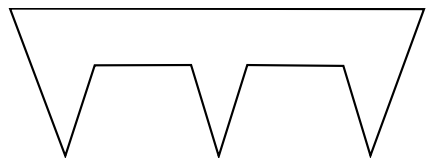Figure 18

Chvátal’s theorem gives the answer: for a gallery with $$n$$ walls, $$\llcorner n/3\lrcorner$$ guards suffice, where $$\llcorner n/3\lrcorner$$ is the greatest integer $$\le n/3$$. (If this does not sound to you sufficiently like a mathematical theorem, it can be restated as follows: Let $$S$$ be a subset of the Euclidean plane. For a subset $$B$$ of $$S$$ let us say that $$B$$ supervises $$S$$ iff for each $$x \in S$$ there is a $$y \in B$$ such that the segment $$xy$$ lies within $$S$$. Then the smallest number $$f(n)$$ such that every set bounded by a simple $$n$$-gon is supervised by a set of $$f(n)$$ points is at most $$\llcorner n/3.\lrcorner$$

Here is Steve Fisk’s proof. A short induction shows that every polygon can be triangulated, i.e., non-crossing edges between non-adjacent vertices (“diagonals”) can be added so that the polygon is entirely composed of non-overlapping triangles. So take any $$n$$-sided polygon with a fixed triangulation. Think of it as a graph, a set of vertices and connected edges, as in Figure 19.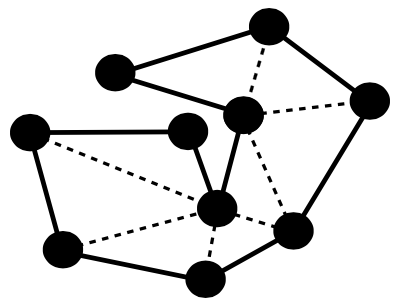Figure 19

The first part of the proof shows that the graph is 3-colourable, i.e., every vertex can be coloured with one of just three colours (red, white and blue, say) so that no edge connects vertices of the same colour.

The argument proceeds by induction on $$n \ge 3$$, the number of vertices.

For $$n = 3$$ it is trivial. Assume it holds for all $$k$$, where $$3 \le k < n$$.

Let triangulated polygon $$G$$ have $$n$$ vertices. Let $$u$$ and $$v$$ be any two vertices connected by diagonal edge $$uv$$. The diagonal $$uv$$ splits $$G$$ into two smaller graphs, both containing $$uv$$. Give $$u$$ and $$v$$ different colours, say red and white, as in Figure 20.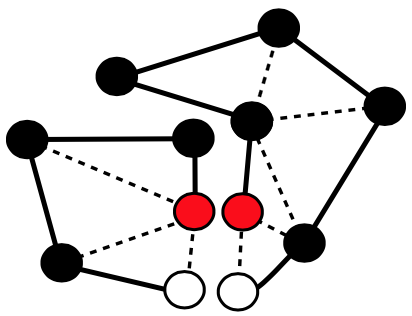Figure 20

By the inductive assumption, we may colour each of the smaller graphs with the three colours so that no edge joins vertices of the same colour, keeping fixed the colours of $$u$$ and $$v$$. Pasting together the two smaller graphs as coloured gives us a 3-colouring of the whole graph.

What remains is to show that $$\llcorner n/3\lrcorner$$ or fewer guards can be placed on vertices so that every triangle is in the view of a guard. Let $$b$$, $$r$$ and $$w$$ be the number of vertices coloured blue, red and white respectively. Let $$b$$ be minimal in $$\{b, r, w\}$$. Then $$b \le r$$ and $$b \le w$$. Then $$2b \le r + w$$. So $$3b \le b + r + w = n$$. So $$b \le n/3$$ and so $$b \le \llcorner n/3\lrcorner$$. Place a guard on each blue vertex. Done.

The central idea of this proof, or the proof strategy, is clear. While the actual diagrams produced here are superfluous to the proof, some visualizing enables us to grasp the central idea.

## 8. Conclusion

Thinking which involves the use of seen or visualized images, which may be static or moving, is widespread in mathematical practice. Such visual thinking may constitute a non-superfluous and non-replaceable part of thinking through a specific proof. But there is a real danger of over-generalisation when using images, which we need to guard against, and in some contexts, such as real and complex analysis, the apparent soundness of a diagrammatic inference is liable to be illusory.

Even when visual thinking does not contribute to proving a mathematical truth, it may enable one to discover a truth, where to discover a truth is to come to believe it in an independent, reliable and rational way. Visual thinking can also play a large role in discovering a central idea for a proof or a proof-strategy; and in discovering a kind of mathematical entity or a mathematical property.

The (non-superfluous) use of visual thinking in coming to know a mathematical truth does in some cases introduce an a posteriori element into the way one comes to know it, resulting in a posteriori mathematical knowledge. This is not as revolutionary as it may sound as a truth knowable a posteriori may also be knowable a priori. More interesting is the possibility that one can acquire some mathematical knowledge in a way in which visual thinking is essential but does not contribute evidence; in this case the role of the visual thinking may be to activate one’s prior cognitive resources. This opens the possibility that non-superfluous visual thinking may result in a priori knowledge of a mathematical truth.

Visual thinking may contribute to understanding in more than one way. Visual illustrations may be extremely useful in providing examples and non-examples of analytic concepts, thus helping to sharpen our grasp of those concepts. Also, visual thinking accompanying a proof may deepen our understanding of the proof, giving us an awareness of the direction of the proof so that, as Hermann Weyl put it, we are not forced to traverse the steps blindly, link by link, feeling our way by touch.

## Bibliography

• Adams, C., 2001, The Knot Book, Providence, Rhode Island: American Mathematical Society.
• Azzouni, J., 2013, “That we see that some diagrammatic proofs are perfectly rigorous”, Philosophia Mathematica, 21: 323–338.
• Brown, J., 1999, Philosophy of Mathematics: an introduction to the world of proofs and pictures, London: Routledge.
• Barwise, J. and J. Etchemendy, 1996, “Visual information and valid reasoning”, in Logical Reasoning with Diagrams, G. Allwein and J. Barwise (eds) Oxford: Oxford University Press.
• Bolzano, B., 1817, “Purely analytic proof of the theorem that between any two values which give results of opposite sign there lies at least one real root of the equation”, in Ewald 1996: vol. 1, 225–248.
• Brooks, N., Barner, D., Frank, M. and Goldin-Meadow, S., 2018, The role of gesture in supporting mental representations: The case of mental abacus arithmetic. Cognitive Science, 45(2), 554–575.
• Carter, J., 2010, “Diagrams and Proofs in Analysis”, International Studies in the Philosophy of Science, 24: 1–14.
• Cauchy, A., 1813, “Recherche sur les polyèdres—premier mémoire”, Journal de l’Ecole Polytechnique, 9: 66–86.
• Chen, F., Hu, Z., Zhao, X., Wang, R. and Tang, X., 2006, Neural correlates of serial abacus mental calculation in children: a functional MRI study. Neuroscience Letters, 403(1–2), 46‐51.
• Chvátal, V., 1975, “A Combinatorial Theorem in Plane Geometry”, Journal of Combinatorial Theory, series B,18: 39–41, 1975.
• Dedekind, R., 1872, “Continuity and the Irrational Numbers”, in Essays on the Theory of Numbers, W. Beman (trans.) New York: Dover Publications.
• De Toffoli, S. and V. Giardino, 2014, “Forms and Roles of Diagrams in Knot Theory”, Erkenntnis, 79: 829–842.
• Eddy, R., 1985, “Behold! The Arithmetic-Geometric Mean Inequality”, College Mathematics Journal, 16: 208. Reprinted in Nelsen 1993: 51.
• Euclid, Elements, Published as Euclid’s Elements: all thirteen books complete in one volume, T. Heath (trans.), D. Densmore (ed.). Santa Fe: Green Lion Press 2002.
• Ewald, W. (ed.), 1996, From Kant to Hilbert. A Source Book in the Foundations of Mathematics, Volumes 1 and 2. Oxford: Clarendon Press.
• Fisk, S., 1978, “A Short Proof of Chvátal’s Watchman Theorem”, Journal of Combinatorial Theory, series B, 24: 374.
• Fomenko, A., 1994, Visual Geometry and Topology, M. Tsaplina (trans.) New York: Springer.
• Frank, M. and Barner, D., 2012, Representing exact number visually using mental abacus. Journal of Experimental Psychology: General, 141(1), 134–149.
• Giaquinto, M., 1993b, “Visualizing in Arithmetic”, Philosophy and Phenomenological Research, 53: 385–396.
• –––, 1994, “Epistemology of visual thinking in elementary real analysis”, British Journal for the Philosophy of Science, 45: 789–813.
• –––, 2007, Visual Thinking in Mathematics, Oxford: Oxford University Press.
• –––, 2011, “Crossing curves: a limit to the use of diagrams in proofs”, Philosophia Mathematica, 19: 281–307.
• Gromov, M., 1993, “Asymptotic invariants of infinite groups”, in Geometric Group Theory, A. Niblo and M. Roller (eds.), LMS Lecture Note Series, Vol. 182, Cambridge: Cambridge University Press, (vol. 2).
• Hahn, H., 1933, “The crisis in intuition”, Translated in Hans Hahn. Empiricism, Logic and Mathematics: Philosophical Papers, B. McGuiness (ed.) Dordrecht: D. Reidel 1980. First published in Krise und Neuaufbau in den exakten Wissenschaften, Fünf Wiener Vorträge, Leipzig and Vienna 1933.
• Hatano, G., Miyake, Y. and Binks, M., 1977, Performance of expert abacus operators. Cognition, 5, 47–55.
• Hilbert, D., 1894, “Die Grundlagen der Geometrie”, Ch. 2, in David Hilbert’s Lectures on the Foundations of Geometry (1891–1902), M. Hallett and U. Majer (eds) Berlin: Springer 2004.
• Hishitani, S., 1990, Imagery experts: How do expert abacus operators process imagery? Applied Cognitive Psychology, 4(1), 33–46.
• Hoffman, D., 1987, “The Computer-Aided Discovery of New Embedded Minimal Surfaces”, Mathematical Intelligencer, 9: 8–21.
• Hu, Y., Geng, F., Tao, L., Hu, N., Du, F., Fu, K. and Chen, F., 2011, Enhanced white matter tracts integrity in children with abacus training. Human Brain Mapping. 32, 10–21.
• Jamnik, M., 2001, Mathematical Reasoning with Diagrams: From Intuition to Automation, Stanford, California: CSLI Publications.
• Joyal, A., R. Street, and D. Verity, 1996, “Traced monoidal categories”, Mathematical Proceedings of the Cambridge Philosophical Society, 119(3) 447–468.
• Kant, I., 1781/9, Kritik der reinen Vernunft, P. Guyer and A. Wood (trans. & eds), Cambridge: Cambridge University Press, 1998.
• Klein, F., 1893, “Sixth Evanston Colloquium lecture”, in The Evanston Colloquium Lectures on Mathematics, New York: Macmillan 1911. Partially reprinted in Ewald 1996: vol. 2: 958-65.
• Kojima, T., 1954, The Japanese abacus: Its use and theory. Tokyo, Japan. Tuttle.
• Landau, E., 1934, Differential and Integral Calculus, Hausner and Davis (trans.), New York: Chelsea 1950.
• Leinster, T., 2004, “Operads in Higher-dimensional Category theory”, Theory and Applications of Categories, 12(3): 73–194.
• Littlewood, J., 1953, “Postscript on Pictures”, in Littlewood’ Miscellany, Cambridge: Cambridge University Press 1986.
• Lyusternik, L., 1963, Convex Figures and Polyhedra, T. Smith (trans.), New York: Dover Publications.
• Mancosu, P., 2005, “Visualization in Logic and Mathematics”, in P. Mancosu, K. Jørgensen and S. Pedersen (eds), Visualization, Explanation and Reasoning Styles in Mathematics, Dordrecht: Springer.
• –––, 2011, “Explanation in Mathematics”, The Stanford Encyclopedia of Philosophy (Summer 2011 Edition), Edward N. Zalta (ed.), URL = <https://plato.stanford.edu/archives/sum2011/entries/mathematics-explanation/>.
• Maxwell, E., 1959, Fallacies in Mathematics, Cambridge University Press.
• Montuchi, P. and W. Page, 1988, “Behold! Two extremum problems (and the arithmetic-geometric mean inequality)”, College Mathematics Journal, 19: 347. Reprinted in Nelsen 1993: 52.
• Miller, N., 2001, A Diagrammatic Formal System for Euclidean Geometry, Ph. D. Thesis, Cornell University.
• Mumma, J. and M. Panza, 2012, “Diagrams in Mathematics: History and Philosophy”, Synthese, 186: Issue 1.
• Needham, T., 1997, Visual Complex Analysis, Oxford: Clarendon Press.
• Nelsen, R., 1993, Proofs Without Words: Exercises in Visual Thinking, Washington DC: The Mathematical Association of America.
• Palais, R., 1999, “The visualization of mathematics: towards a mathematical exploratorium”, Notices of the American Mathematical Society, 46: 647–658.
• Pasch, M., 1882, Vorlesungen über neuere Geometrie, Berlin: Springer 1926, 1976 (with introduction by Max Dehn).
• Rouse Ball, W., 1939, Mathematical Recreations and Essays, Revised by H. Coxeter, 11th edition. (First published in 1892). New York: Macmillan.
• Russell, B., 1901, “Recent Work on the Principles of Mathematics”, International Monthly, 4: 83–101. Reprinted as “Mathematics and the Metaphysicians” in Mysticism and Logic, London: George Allen and Unwin 1918.
• Shin, Sun-Joo, Oliver Lemon, and John Mumma, 2013, “Diagrams”, The Stanford Encyclopedia of Philosophy (Fall 2013 Edition), Edward N. Zalta (ed.), URL = <https://plato.stanford.edu/archives/fall2013/entries/diagrams/>.
• Starikova, I., 2012, “From Practice to New Concepts: Geometric Properties of Groups”, Philosophia Scientiae, 16(1): 129–151.
• Stigler, J., 1984, “Mental Abacus”: The effect of abacus training on Chinese children's mental calculation. Cognitive Psychology, 16, 145–176.
• Tappenden, J., 2005, “Proof style and understanding in mathematics I: visualization, unification and axiom choice”, in Mancosu, P., Jørgensen, K. and Pedersen, S. (eds) Visualization, Explanation and Reasoning Styles in Mathematics, Dordrecht: Springer.
• Tennant, N., 1986, “The Withering Away of Formal Semantics?” Mind and Language, 1(4): 382–318.
• Van den Dries, L., 1998, Tame Topology and O-minimal Structures, LMS Lecture Note Series 248, Cambridge University Press.
• Weyl, H., 1995 , “Topology and abstract algebra as two roads of mathematical comprehension”, American Mathematical Monthly, 435–460 and 646–651. Translated by A. Shenitzer from an article of 1932, Gesammelte Abhandlungen, 3: 348–358.
• Zimmermann W. and S. Cunningham (eds), 1991,Visualization in Teaching and Learning Mathematics, Washington, DC: Mathematical Association of America.How to cite this entry.Preview the PDF version of this entry at the Friends of the SEP Society.Look up topics and thinkers related to this entry at the Internet Philosophy Ontology Project (InPhO).Enhanced bibliography for this entry at PhilPapers, with links to its database.